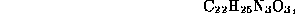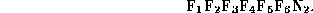Next: References Up: MOLGEN, a generator of... Previous: The use of

# The use of MOLGEN in research

Here is an interesting example: People from BASF, Germany, gave us the following problem: The chemical formula isand we were told that the molecule in question contains the following 6 nonoverlapping substructures (macroatoms, as they were described above):Using these substructures, the gross formula reduces toMOLGEN obtains (after several interactive steps which take into account further properties of the chemical substance in question, and which we are not going to describe in detail here for lack of space) 2,337 constitutional formulae for that reduced gross formula, and after expansion it constructs 8,916 isomers. Now we used the following badlist: no triple bonds and ring sizes only between 5 and 6 were allowed, so that there remained 201 candidates only from which an expert easily obtained the correct solution, which turned out to be the following one among these candidates:Send questions to: molgen@btm2x2.mat.uni-bayreuth.de